# Affichage¶

In :
Sys.interactive := false;
Topdirs.dir_directory (Sys.getenv "OCAML_TOPLEVEL_PATH");

#use "topfind"
#require "jupyter.notebook";;

let graphviz str = (* C'est moche mais ça fonctionne *)
let filename name = (Unix.getenv "HOME")^"/"^name in (* Unix only *)
let dot_file = filename "tmp-ocaml.dot" and png_file = filename "tmp-ocaml.png" in
let oc = open_out dot_file in Printf.fprintf oc "%s\n" str; close_out oc;
(* ignore (Sys.command ("dot "^dot_file^" -Tpng -o "^png_file)); *)
(* dot input.dot | gvpr -c -f tree.gv | neato -n -Tpdf -o output.pdf *)
ignore (Sys.command ("dot "^dot_file^" | gvpr -c -f tree-ljouhet.gv | neato -n -Tpng -o "^png_file));
ignore (Jupyter_notebook.display_file ~base64:true "image/png" png_file)

let walk next defaults a =
let s = ref [] and cpt = ref 0 in
let rec aux cpt_parent noeud =
incr cpt;
let cpt_self = !cpt in
let suivants, node_props, edge_props = next noeud in
let props = (String.concat " " (List.map (fun (a, b) -> Printf.sprintf "%s=\"%s\"" a b) node_props)) in
s := (Printf.sprintf "%d [%s]" cpt_self props)::!s;
List.iter (aux cpt_self) suivants;
let props = (String.concat " " (List.map (fun (a, b) -> Printf.sprintf "%s=\"%s\"" a b) edge_props)) in
s := (Printf.sprintf "%d -> %d [%s]" cpt_parent cpt_self props)::!s;
in aux 0 a;
String.concat "\n" (["digraph G {"]@defaults@["0 [shape=point style=invis]"]@(List.rev !s)@["}"])

let make_affiche next defaults x = graphviz (walk next defaults x)

Out:
- : unit = ()

- : unit = ()
- : unit = ()

Out:
val graphviz : string -> unit = <fun>

Out:
val walk :
('a -> 'a list * (string * string) list * (string * string) list) ->
string list -> 'a -> string = <fun>

Out:
val make_affiche :
('a -> 'a list * (string * string) list * (string * string) list) ->
string list -> 'a -> unit = <fun>


# Arbres Rouge-Noir¶

In :
(* Source : https://www.cs.cornell.edu/courses/cs3110/2009sp/lectures/src/lec11.ml *)

(* Red-Black tree.  Binary search tree with properties:
* 1. Nodes are colored either red or black.
* 2. The root is black
* 3. Every leaf is black.
* 4. The children of every red node are black.
* 5. Every path from a node to a descendant leaf has the same
*    number of black nodes as every other path.
*
* Longest path from root to leaf can be at most 2x longer than
* shortest path because at most every other node can be red (no
* red node with red parents) and same number of black nodes on
* each path, thus search always O(log n).
*)

type color = Red | Black

type 'a rbtree = Node of color * 'a * 'a rbtree * 'a rbtree | Leaf

(* Affichage *)
let next_rb x = match x with
| Leaf -> [], [("style", "invis")], [("style", "invis")]
| Node (c, i, a, b) -> let couleur = if c = Red then "#ff0000" else "#000000" in
[a; b], [("label", string_of_int i);("fillcolor", couleur)], []

let affiche = make_affiche next_rb ["node [shape=circle fontcolor=\"#ffffff\" style=filled]"]

let rec mem x = function
Leaf -> false
| Node (_, y, left, right) -> x = y || (x < y && mem x left) || (x > y && mem x right)

(* Repair violations of the invariant that a red node should have no
* red children.  Make this the responsibility of the grandparent of
* the red node having the red parent.  There are four cases each of
* which results in a red node with two black children (which may in
* turn violate the constraint above it in the tree.  *)

let balance = function
Black, z, Node (Red, y, Node (Red, x, a, b), c), d
| Black, z, Node (Red, x, a, Node (Red, y, b, c)), d
| Black, x, a, Node (Red, z, Node (Red, y, b, c), d)
| Black, x, a, Node (Red, y, b, Node (Red, z, c, d)) ->
Node (Red, y, Node (Black, x, a, b), Node (Black, z, c, d))
| a, b, c, d ->
Node (a, b, c, d)

let insert x s =
(* same as for basic binary search tree except 3 things:
* 1. initially replace a leaf with a red node with two leaves as
* children, 2. balance result of each recursive call (because
* inserting red node may have violated red has no red children
* invariant), 3. force root to be black
*
* Note considerably simpler than imperative version of insert, which
* has left and right rotations in addition to operation similar to
* balance's grandparent update. *)
let rec ins = function
Leaf -> Node (Red, x, Leaf, Leaf)
| Node (color, y, a, b) as s ->
if x < y then balance (color, y, ins a, b)
else if x > y then balance (color, y, a, ins b)
else s
in
match ins s with
Node (_, y, a, b) ->
Node (Black, y, a, b)
| Leaf -> (* guaranteed to be nonempty *)
raise (Failure "RBT insert failed with ins returning leaf")

let rec rbt_of_list = function
| [] -> Leaf
| x :: l -> insert x (rbt_of_list l);;

Out:
type color = Red | Black

Out:
type 'a rbtree = Node of color * 'a * 'a rbtree * 'a rbtree | Leaf

Out:
val next_rb :
int rbtree ->
int rbtree list * (string * string) list * (string * string) list = <fun>

Out:
val affiche : int rbtree -> unit = <fun>

Out:
val mem : 'a -> 'a rbtree -> bool = <fun>

Out:
val balance : color * 'a * 'a rbtree * 'a rbtree -> 'a rbtree = <fun>

Out:
val insert : 'a -> 'a rbtree -> 'a rbtree = <fun>

Out:
val rbt_of_list : 'a list -> 'a rbtree = <fun>


# Exemples¶

In :
affiche (rbt_of_list [12; 3; 7; 1; 21; 7; 23; 2; 8])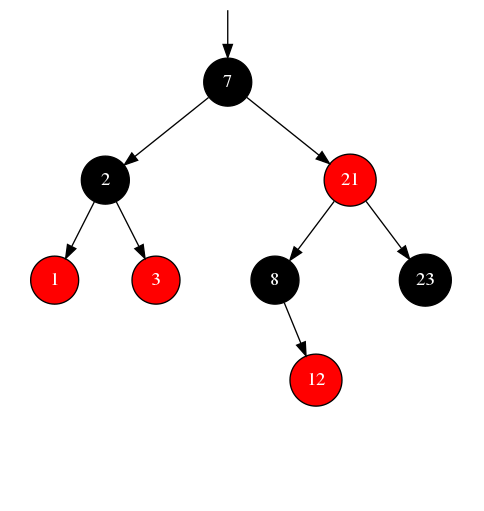Out:
- : unit = ()

In :
let rec range a b =
if a >= b then []
else a::range (a+1) b

Out:
val range : int -> int -> int list = <fun>

In :
affiche (rbt_of_list (range 0 30))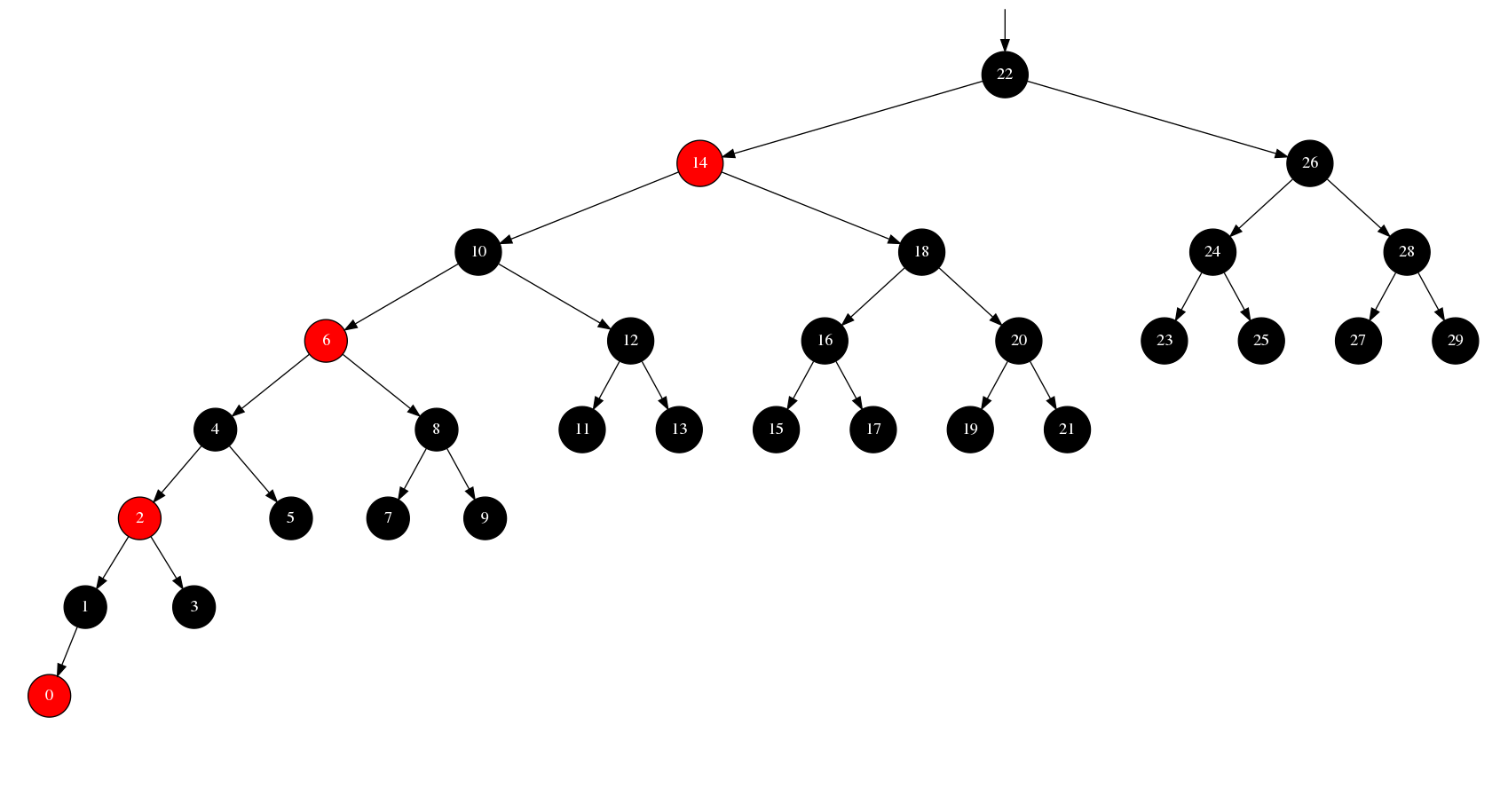Out:
- : unit = ()

In :
let shuffle arr =
for n = Array.length arr - 1 downto 1 do
let k = Random.int (n + 1) in
let temp = arr.(n) in
arr.(n) <- arr.(k);
arr.(k) <- temp
done;
arr

Out:
val shuffle : 'a array -> 'a array = <fun>

In :
for i = 1 to 5 do
let items = Array.to_list (shuffle (Array.init 15 (fun x->x))) in
affiche (rbt_of_list items)
done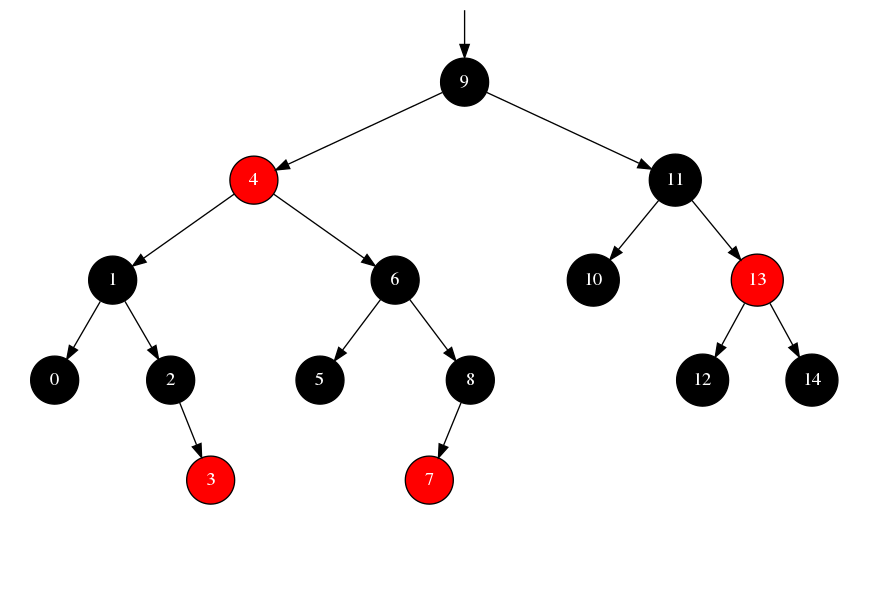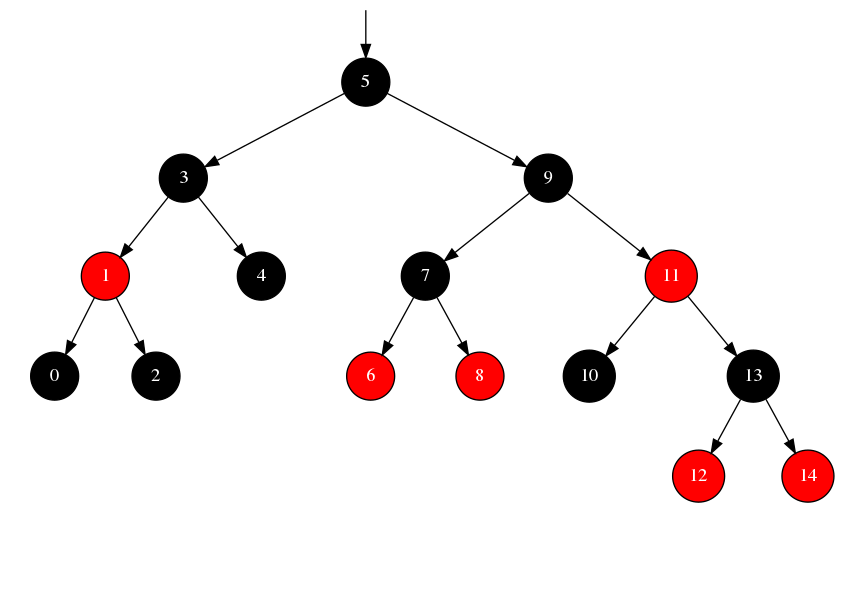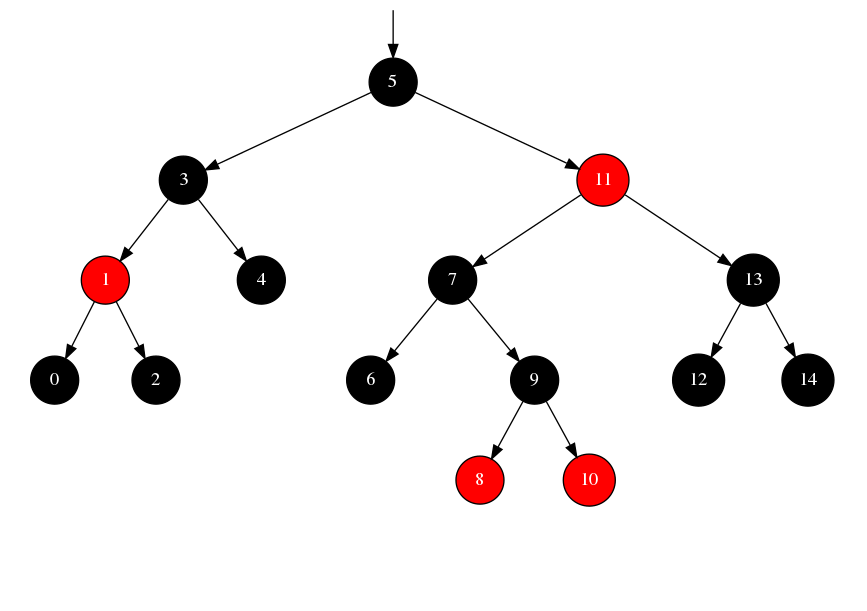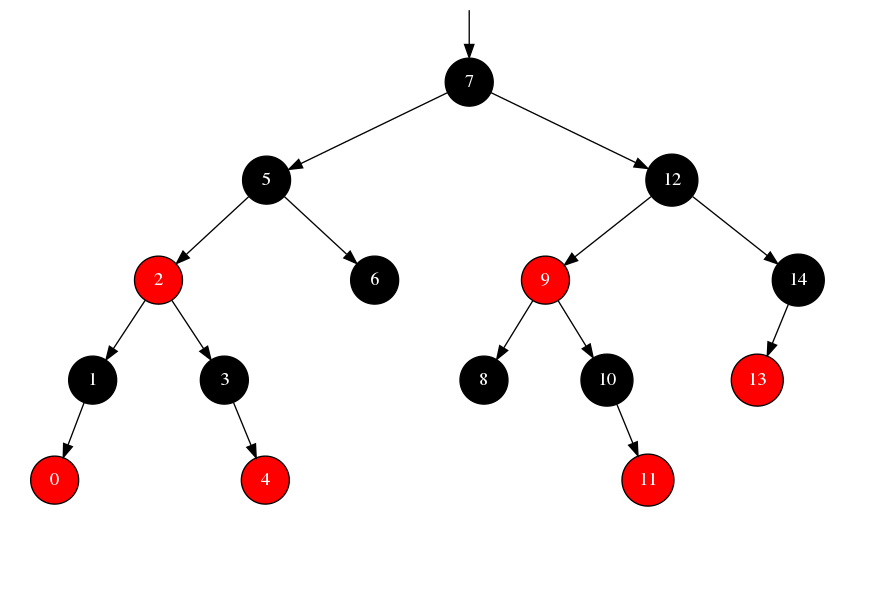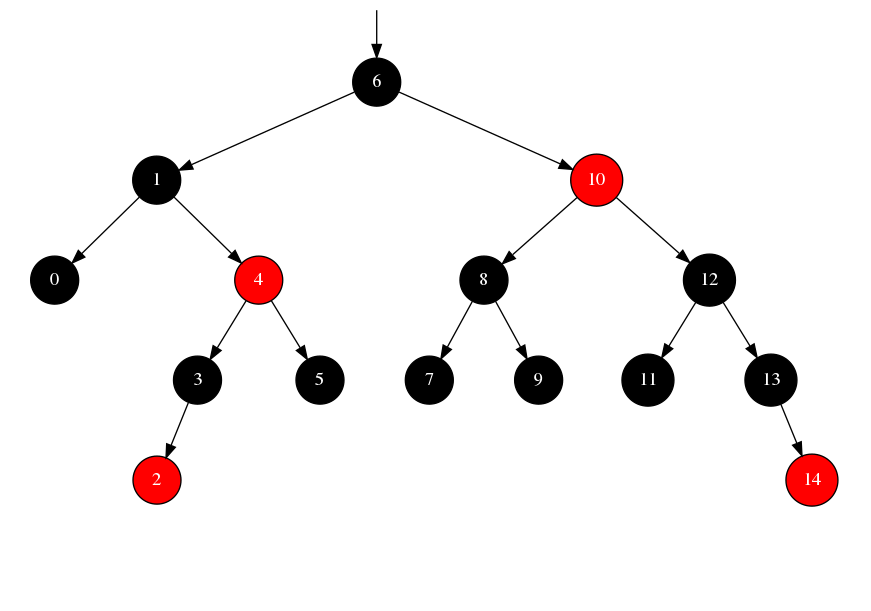Out:
- : unit = ()

In :
let arbre = ref Leaf;;

Out:
val arbre : '_a rbtree ref = {contents = Leaf}

In :
arbre := insert (Random.int 100) !arbre;
affiche !arbre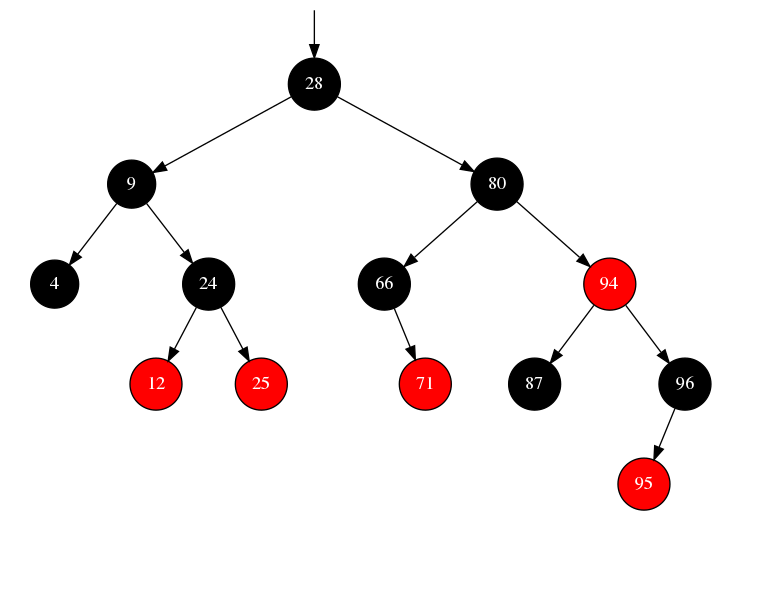Out:
- : unit = ()

In [ ]:


In [ ]:


In [ ]:


In [ ]:


In [ ]:


In [ ]:


In [ ]:


In [ ]:


In [ ]:


In [ ]:


In [ ]:


In [ ]:


In [ ]:


In [ ]:


In [ ]:


In [ ]: